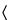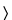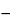Sodium Bicarbonate
(soe' dee um bye kar' bo nate).

NaHCO384.01
Carbonic acid monosodium salt;
Monosodium carbonate[144-55-8].
DEFINITION
Sodium Bicarbonate contains NLT 99.0% and NMT 100.5% of NaHCO3, calculated on the dried basis.
IDENTIFICATION
ASSAY
•  Procedure
Sample: 3 g
Analysis: Mix the Sample with 100 mL of water, add methyl red TS, and titrate with 1 N hydrochloric acid VS. Add the acid slowly, with constant stirring, until the solution becomes faintly pink. Heat the solution to boiling, cool, and continue the titration until the faint pink color no longer fades after boiling. Each mL of 1 N hydrochloric acid is equivalent to 84.01 mg of sodium bicarbonate.
Acceptance criteria: 99.0%–100.5% on the dried basis
IMPURITIES
•  Insoluble Substances
Sample solution: Dissolve 1 g in 20 mL of water.
Acceptance criteria: The resulting solution is complete and clear.
•  Carbonate (where it is labeled as intended for use in hemodialysis)
Apparatus: The apparatus (see Figure 1) consists of a 50-mL flask with a side arm connected to a source of carbon dioxide humidified by bubbling through a saturated solution of sodium bicarbonate. The flask is equipped with a top-mounted stopper fitted with an exit tube. The exit tube is connected via a T-tube to a system vent and a leveling buret and reservoir.
Reagents
Saturated sodium bicarbonate solution: Mix 20 g of sodium bicarbonate in 100 mL of water, and allow any undissolved crystals to settle. Use the clear supernatant.
Displacement solution: Dissolve 100 g of sodium chloride in 350 mL water, and add 1 g of sodium bicarbonate and 1 mL of methyl orange TS. After the sodium bicarbonate has dissolved, add 6 N sulfuric acid until the solution turns pink. Use this solution to fill the reservoir of the apparatus.
Determine the total volume, V, in mL, of carbon dioxide absorbed after the addition of the specimen to the flask, and calculate the percentage of carbonate in the portion of specimen tested:
Result = 273V × (6001P)/[22400 × (273 + T) × (760W)]
 V = = total volume of carbon dioxide absorbed (mL) P = = ambient atmospheric pressure (mm of mercury) T = = ambient temperature W = = weight of Sodium Bicarbonate taken (g)
[Note—Maintain a constant temperature during the measurement of the volume of carbon dioxide absorbed. ]
Acceptance criteria: NMT 0.23%
•  Normal Carbonate
Sample: 1 g
Analysis:  Dissolve the Sample in 20 mL of water with very gentle swirling at a temperature not exceeding 15. Add 2.0 mL of 0.10 N hydrochloric acid and 2 drops of phenolphthalein TS.
Acceptance criteria: The solution does not assume more than a faint pink color immediately.
Sample: 0.35 g
Acceptance criteria: No more chloride than corresponds to 1.48 mL of 0.0010 N hydrochloric acid (NMT 0.015%).
•  Limit of Sulfur Compounds
Sample: 2.0 g
Sample solution: Dissolve the Sample in 20 mL of water, and evaporate to 5 mL by boiling. Add 1 mL of bromine TS, evaporate to dryness, and cool. Dissolve the residue in 10 mL of 3 N hydrochloric acid, evaporate to dryness, and cool. Dissolve the residue in 5 mL of 3 N hydrochloric acid, evaporate to dryness, and cool. Dissolve the residue in 10 mL of water, and adjust with 3 N hydrochloric acid or 6 N ammonium hydroxide to a pH of 2. If necessary to obtain a clear solution, filter the solution, washing the filter with two 2-mL portions of water. Dilute with water to 20 mL.
Standard solution: To 0.30 mL of 0.020 N sulfuric acid add 1 mL of 0.06 N hydrochloric acid, and dilute with water to 20 mL.
Analysis: Add 1 mL of barium chloride TS to each of the Sample solution and the Standard solution. Mix, and allow to stand for 30 min.
Acceptance criteria: Any turbidity produced in the Sample solution is not more intense than that produced in the Standard solution (NMT 0.015%).
•  Aluminum206(where it is labeled as intended for use in hemodialysis)
Test preparation: Transfer 1.0 g to a 100-mL plastic volumetric flask. Carefully add 4 mL of nitric acid. Sonicate for 30 min, and dilute with water to volume.
Acceptance criteria: NMT 2 µg/g
Test preparation: Dissolve 1.5 g in 20 mL of 7 N sulfuric acid and add 35 mL of water.
Analysis: Proceed as directed in the chapter, omitting the addition of 20 mL of 7 N sulfuric acid.
Acceptance criteria: NMT 2 ppm
•  Calcium (where it is labeled as intended for use in hemodialysis)
[Note—The Standard solutions and the Sample solution may be modified, if necessary, to obtain solutions of suitable concentrations, adaptable to the linear or working range of the instrument. ]
Potassium chloride solution: 10 mg/mL of potassium chloride in 0.36 N hydrochloric acid
Standard solutions: Transfer 249.7 mg of calcium carbonate, previously dried at 300for 3 h and cooled in a desiccator for 2 h, to a 100-mL volumetric flask. Dissolve in 6 mL of 6 N hydrochloric acid, add 1 g of potassium chloride, and dilute with water to volume. Transfer 10.0 mL of this solution to a second 100-mL volumetric flask, and dilute with Potassium chloride solution to volume. This solution contains 100 µg/mL of calcium. Transfer 2.0, 3.0, 4.0, and 5.0 mL portions of this solution to separate 100-mL volumetric flasks (each containing 6 mL of 6 N hydrochloric acid), and dilute with Potassium chloride solution to volume. These Standard solutions contain 2.0-, 3.0-, 4.0-, and 5.0-µg/mL of calcium, respectively.
Sample solution: Transfer 3.0 g to a 100-mL volumetric flask. Add 6 mL of 6 N hydrochloric acid and 1 g of potassium chloride, and dilute with water to volume.
Blank: Potassium chloride solution
Instrumental conditions
Mode: Atomic absorption spectrophotometry
Analytical wavelength: Calcium emission line at 422.7 nm
Lamp: Calcium hollow-cathode
Flame: Nitrous oxide–acetylene
Analysis
Samples: Standard solutions and Sample solution
Plot the absorbances of the Standard solutions versus their contents of calcium, in µg/mL, by drawing a straight line best fitting the four plotted points. From the graph so obtained determine the concentration, C, in µg/mL, of calcium in the Sample solution.
Calculate the percentage of calcium in the portion of Sodium Bicarbonate taken:
Result = (C/CU) × 100
 C = = concentration of calcium in the Sample solution (µg/mL) CU = = concentration of Sodium Bicarbonate in the Sample solution (µg/mL)
Acceptance criteria: NMT 0.01%
•  Magnesium (where it is labeled as intended for use in hemodialysis)
[Note—The Standard solutions and the Sample solution may be modified, if necessary, to obtain solutions of suitable concentrations, adaptable to the linear or working range of the instrument. ]
Potassium chloride solution, Sample solution, and Blank: Proceed as directed in the test for Calcium.
Standard solutions: To 1.000 g of magnesium in a 250-mL beaker containing 20 mL of water, carefully add 20 mL of hydrochloric acid, warming if necessary to complete the reaction. Transfer this solution to a 1000-mL volumetric flask containing 10 g of potassium chloride, and dilute with water to volume. Transfer 10.0 mL of this solution to a 100-mL volumetric flask containing 1 g of potassium chloride, and dilute with water to volume. Transfer 10.0 mL of this solution to a second 100-mL volumetric flask, and dilute with Potassium chloride solution to volume. This solution contains 10.0 µg/mL of magnesium. Transfer 2.0-, 3.0-, 4.0-, and 5.0-mL portions of this solution to separate 100-mL volumetric flasks (each containing 6 mL of 6 N hydrochloric acid), and dilute with Potassium chloride solution to volume. These Standard solutions contain 0.2, 0.3, 0.4, and 0.5 µg/mL of magnesium, respectively.
Instrumental conditions
Mode: Atomic absorption spectrophotometry
Analytical wavelength: Magnesium emission line at 285.2 nm
Lamp: Magnesium hollow-cathode
Flame: Reducing air–acetylene
Analysis
Samples:  Standard solutions and Sample solution
Plot the absorbances of the Standard solutions versus their contents of magnesium, in µg/mL, by drawing a straight line best fitting the four plotted points. From the graph so obtained determine the concentration, C, in µg/mL, of magnesium in the Sample solution.
Calculate the percentage of magnesium in the portion of Sodium Bicarbonate taken:
Result = (C/CU) × 100
 C = = concentration of magnesium in the Sample solution (µg/mL) CU = = concentration of Sodium Bicarbonate in the Sample solution (µg/mL)
Acceptance criteria: NMT 0.004%
•  Copper (where it is labeled as intended for use in hemodialysis)
[Note—The Standard solution and the Sample solution may be modified, if necessary, to obtain solutions of suitable concentrations, adaptable to the linear or working range of the instrument. ]
Diluent: Dilute 40 mL of nitric acid with water to 1000 mL.
Standard solution: 10.0 µg/mL of copper, prepared as follows. Transfer 1.000 g of copper to a 1000-mL volumetric flask. Dissolve in 20 mL of nitric acid, and dilute with 0.2 N nitric acid to volume. Transfer 10.0 mL of this solution to a second 1000-mL volumetric flask, and dilute with 0.2 N nitric acid to volume. Store in a polyethylene bottle.
Sample solution: Transfer 5.0 g of Sodium Bicarbonate to a 100-mL plastic volumetric flask, and carefully add 4 mL of nitric acid. Sonicate for 30 min, and dilute with water to volume.
Spiked sample solution: To 10.0 mL of the Sample solution add 20 µL of Standard solution. This solution contains 0.02 µg/mL of added copper.
Blank: Diluent
Instrumental conditions
Mode: Atomic absorption spectrophotometry
Analytical wavelength: Copper emission line at 324.7 nm
Lamp: Copper hollow-cathode
Flame: Flameless electrically heated furnace
Analysis
Samples: Sample solution and Spiked sample solution
Plot the absorbances of the Sample solution and the Spiked sample solution versus their contents of added copper, in µg/mL. Draw a line connecting the two points, and extrapolate the line until it intercepts the concentration axis. From the intercept, determine the concentration, C, in µg/mL, of copper in the Sample solution.
Calculate the percentage of copper in the portion of Sodium Bicarbonate taken:
Result = (C/CU) × 100
 C = = concentration of copper in the Sample solution (µg/mL) CU = = concentration of Sodium Bicarbonate in the Sample solution (µg/mL)
Acceptance criteria: NMT 1 ppm
•  Iron241(where it is labeled as intended for use in hemodialysis)
Sample solution: Place 2.0 g of Sodium Bicarbonate in a beaker, and neutralize with hydrochloric acid, noting the volume of acid consumed. Transfer this solution to a 25-mL volumetric flask with the aid of water.
Standard solution: Transfer 1.0 mL of Standard Iron Solution to a 25-mL volumetric flask. Add the same volume of hydrochloric acid as used to prepare the Sample solution.
Blank: Use the same volume of hydrochloric acid in a 25-mL volumetric flask as used to prepare the Sample solution.
Instrumental conditions
Mode: UV-Vis
Analytical wavelength: 480 nm
Analysis
Samples: Sample solution, Standard solution, and Blank
To each of the flasks containing the Standard solution, the Sample solution, and the Blank add 50 mg of ammonium peroxydisulfate crystals and 2 mL of Ammonium Thiocyanate Solution. Dilute with water to volume. Concomitantly determine the absorbances of the solutions from the Standard solution and the Sample solution.
Acceptance criteria: The absorbance of the Sample solution is NMT that of the Standard solution (NMT 5 ppm).
Test preparation: Mix 4.0 g with 5 mL of water and 19 mL of 3 N hydrochloric acid, heat to boiling, and maintain that temperature for 1 min. Add 1 drop of phenolphthalein TS, then add sufficient 6 N ammonium hydroxide, dropwise, to give the solution a faint pink color. Cool, and dilute with water to 25 mL.
Acceptance criteria: NMT 5 ppm
•  Limit of Ammonia
Sodium hypochlorite solution: Use a commercially available solution that contains 4.0%–6.0% of sodium hypochlorite.
Oxidizing solution: Alkaline sodium citrate TS and Sodium hypochlorite solution (4:1). [Note—Prepare on the day of use. ]
Diluted sodium nitroferricyanide solution: Sodium nitroferricyanide TS and water (1:10)
Sample solution: 25 mg/mL in water
Analysis: [Note—Carefully follow the order of addition stated below. ] To 4.0 mL of the Sample solution add 0.4 mL of phenol TS, 0.4 mL of Diluted sodium nitroferricyanide solution, and 1.0 mL of Oxidizing solution. Dilute with water to 10 mL, and allow to stand for 1 h.
Acceptance criteria: No blue color develops.
•  Limit of Organics (where it is labeled as intended for use in hemodialysis)
Silver sulfate solution: 11 g/L of silver sulfate in sulfuric acid
Indicator solution: 14.85 mg/mL of 1,10-phenanthroline and 6.95 mg/mL of ferrous sulfate in water
Standard solution: Transfer 850.3 mg of potassium biphthalate, previously crushed lightly and dried at 120for 2 h, to a 1000-mL volumetric flask. Dilute with water to volume. Transfer 6.0 mL of this solution to a 100-mL volumetric flask, and dilute with water to volume. It contains the equivalent of 0.06 mg/mL of organic equivalents. Transfer 40.0 mL of this solution to a 500-mL reflux flask.
Sample solution: Transfer 20 g of Sodium Bicarbonate to a 500-mL reflux flask. Add 20 mL of water, and swirl. Cautiously add 20 mL of sulfuric acid, and swirl.
[Caution—Perform this operation under a hood. ]
Analysis
Samples: Standard solution, Sample solution, and Blank
To verify the suitability of the system, calculate the amount, in mg, of organic equivalents in the Standard solution:
Result = (VBVS) × 8N
 VB = = volume of 0.07 N ferrous ammonium sulfate VS consumed by the Blank (mL) VS = = volume of 0.07 N ferrous ammonium sulfate VS consumed by the Standard solution (mL) N = = normality of the ferrous ammonium sulfate VS
Suitability criteria: 2.328–2.424 mg
Calculate the amount, in mg, of organic equivalents in the portion of Sodium Bicarbonate taken:
Result = (VBVU) × 8N
 VB = = volume of 0.07 N ferrous ammonium sulfate VS consumed by the Blank (mL) VU = = volume of 0.07 N ferrous ammonium sulfate VS consumed by the Sample solution (mL) N = = normality of the ferrous ammonium sulfate VS
Acceptance criteria: NMT 0.01%
SPECIFIC TESTS
Dry 4 g over silica gel for 4 h: it loses NMT 0.25% of its weight.